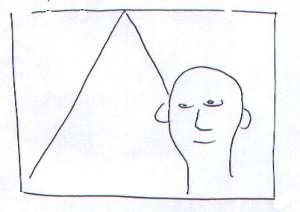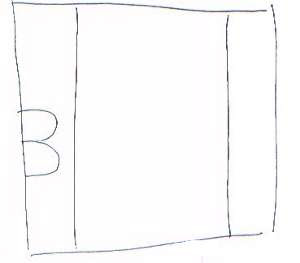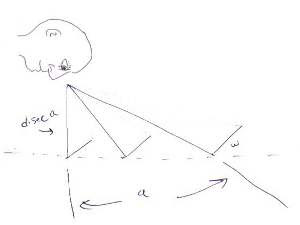## Sunday, March 21, 2010

### Curvilinear Perspective

A while back I noticed a problem with linear perspective. In linear perspective, a road vanishes to a single point on the horizon:However, this would be the case in both directions the person is looking. That would mean that if a person looked straight down, and linear perspective really described how objects appear to shrink, the observer ought to see something like this:However, of course, what the observer sees are two parallel lines:This means the that the lines are actually curved, since they join at both ends. But what is this curve?

First we must consider why would an object look smaller when it's further away? The apparent size is determined by the angle that's subtended with the eyes. Moving a object further away creates a smaller apparent angle.Applying a little basic trigonometry, the tangent of the apparent angle formed between the road and eye would be:

tan(size/2) = width / (distance * sec (a)) = cos(a) * width / distance

So:

size = 2 * arctan[ cos(a) * width / distance ]

Where "a" is the viewing angle, and "size" is the size it appears to be. This means the curve of the road might look something like:Which explains why linear perspective is a great approximation for perspective, even though it isn't always usable. About 1/3 on each end is approximately a straight line, and the middle 1/3 is curved slightly.